# 8. Rate of return analysis

16 Mar 2015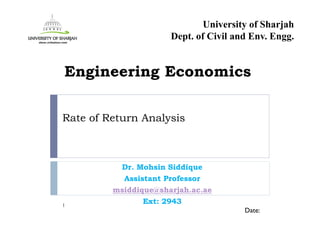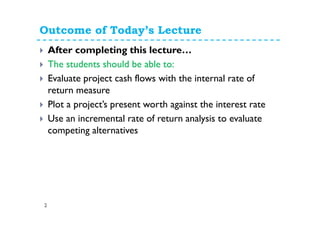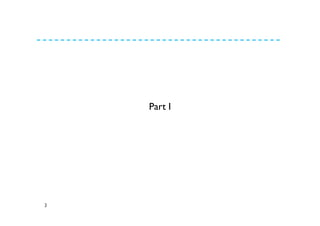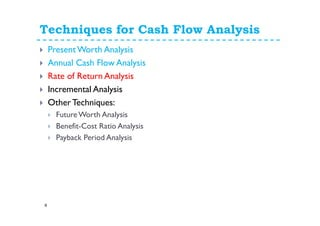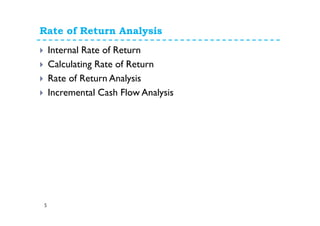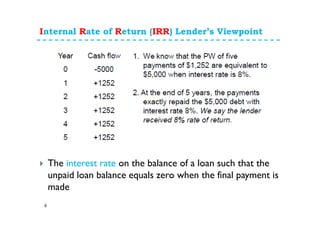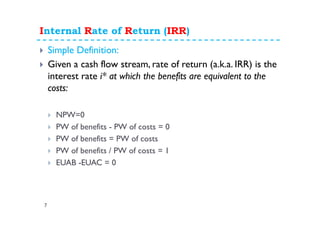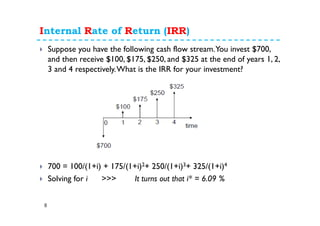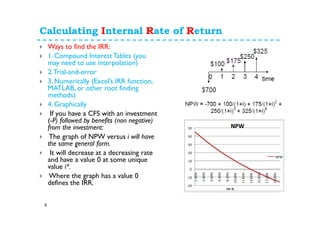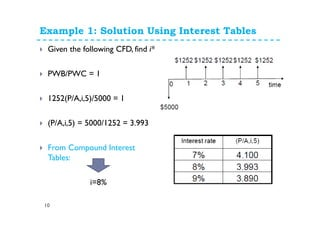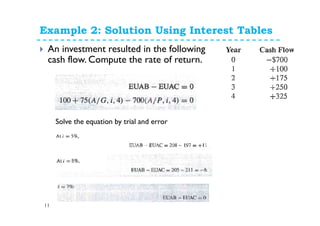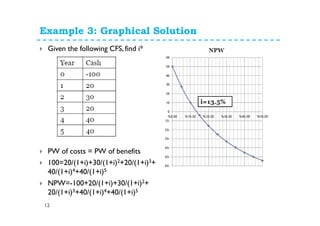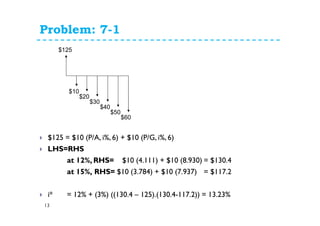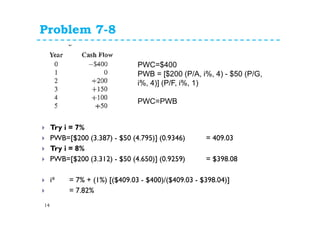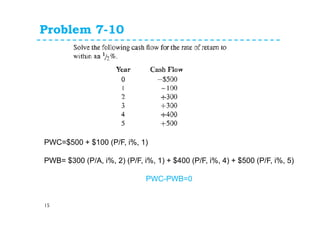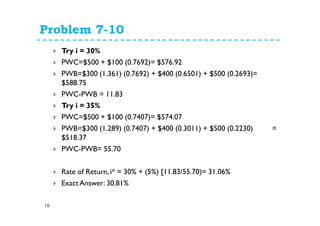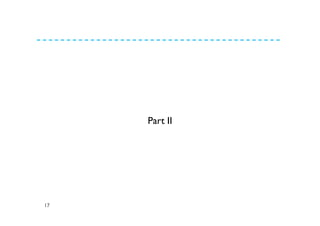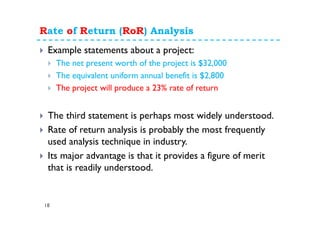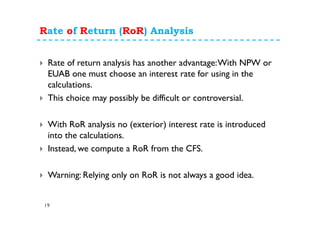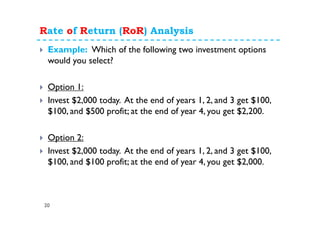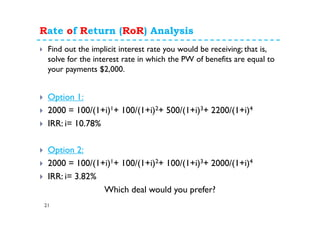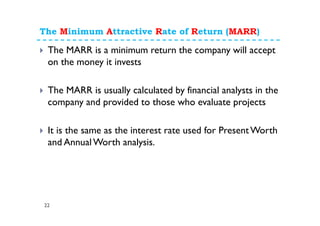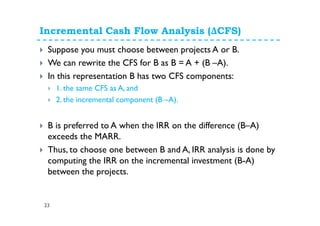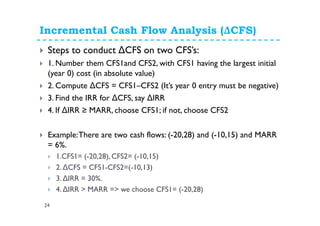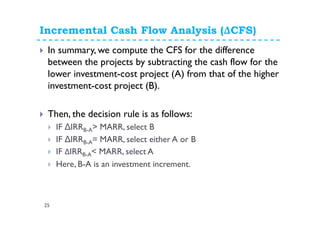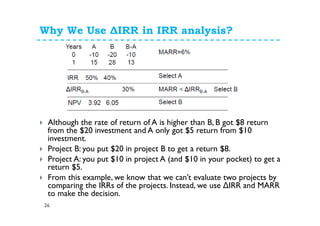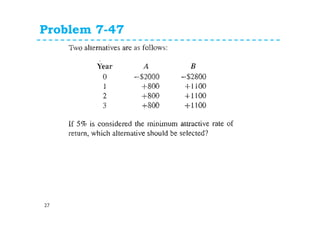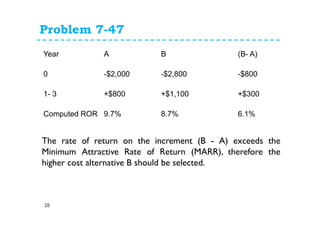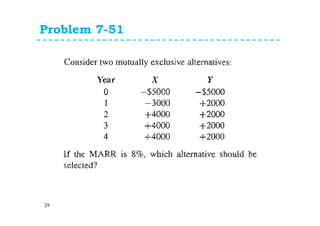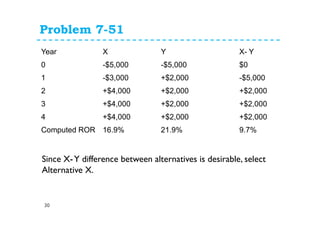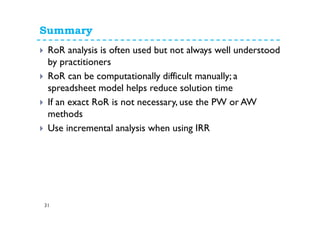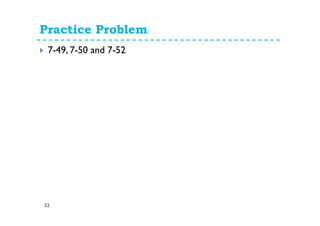1 sur 33

### 8. Rate of return analysis

• 1. Rate of Return Analysis Dr. Mohsin Siddique Assistant Professor msiddique@sharjah.ac.ae Ext: 29431 Date: Engineering Economics University of Sharjah Dept. of Civil and Env. Engg.
• 2. Outcome of Today’s Lecture 2 After completing this lecture… The students should be able to: Evaluate project cash flows with the internal rate of return measure Plot a project’s present worth against the interest rate Use an incremental rate of return analysis to evaluate competing alternatives
• 3. 3 Part I
• 4. Techniques for Cash Flow Analysis 4 Present Worth Analysis Annual Cash Flow Analysis Rate of Return Analysis Incremental Analysis OtherTechniques: FutureWorth Analysis Benefit-Cost Ratio Analysis Payback Period Analysis
• 5. Rate of Return Analysis 5 Internal Rate of Return Calculating Rate of Return Rate of Return Analysis Incremental Cash Flow Analysis
• 6. Internal Rate of Return (IRR) Lender’s Viewpoint 6 The interest rate on the balance of a loan such that the unpaid loan balance equals zero when the final payment is made
• 7. Internal Rate of Return (IRR) 7 Simple Definition: Given a cash flow stream, rate of return (a.k.a. IRR) is the interest rate i* at which the benefits are equivalent to the costs: NPW=0 PW of benefits - PW of costs = 0 PW of benefits = PW of costs PW of benefits / PW of costs = 1 EUAB -EUAC = 0
• 8. Internal Rate of Return (IRR) 8 Suppose you have the following cash flow stream.You invest \$700, and then receive \$100, \$175, \$250, and \$325 at the end of years 1, 2, 3 and 4 respectively.What is the IRR for your investment? 700 = 100/(1+i) + 175/(1+i)2+ 250/(1+i)3+ 325/(1+i)4 Solving for i >>> It turns out that i* = 6.09 %
• 9. Calculating Internal Rate of Return 9 Ways to find the IRR: 1. Compound InterestTables (you may need to use interpolation) 2.Trial-and-error 3. Numerically (Excel’s IRR function, MATLAB, or other root finding methods) 4. Graphically If you have a CFS with an investment (-P) followed by benefits (non negative) from the investment: The graph of NPW versus i will have the same general form. It will decrease at a decreasing rate and have a value 0 at some unique value i*. Where the graph has a value 0 defines the IRR.
• 10. Example 1: Solution Using Interest Tables 10 Given the following CFD, find i* PWB/PWC = 1 1252(P/A,i,5)/5000 = 1 (P/A,i,5) = 5000/1252 = 3.993 From Compound Interest Tables: i=8%
• 11. Example 2: Solution Using Interest Tables 11 An investment resulted in the following cash flow. Compute the rate of return. Solve the equation by trial and error
• 12. Example 3: Graphical Solution 12 Given the following CFS, find i* PW of costs = PW of benefits 100=20/(1+i)+30/(1+i)2+20/(1+i)3+ 40/(1+i)4+40/(1+i)5 NPW=-100+20/(1+i)+30/(1+i)2+ 20/(1+i)3+40/(1+i)4+40/(1+i)5
• 13. Problem: 7-1 13 \$125 = \$10 (P/A, i%, 6) + \$10 (P/G, i%, 6) LHS=RHS at 12%, RHS= \$10 (4.111) + \$10 (8.930) = \$130.4 at 15%, RHS= \$10 (3.784) + \$10 (7.937) = \$117.2 i* = 12% + (3%) ((130.4 – 125).(130.4-117.2)) = 13.23% \$125 \$10 \$20 \$30 \$40 \$50 \$60
• 14. Problem 7-8 14 Try i = 7% PWB=[\$200 (3.387) - \$50 (4.795)] (0.9346) = 409.03 Try i = 8% PWB=[\$200 (3.312) - \$50 (4.650)] (0.9259) = \$398.08 i* = 7% + (1%) [(\$409.03 - \$400)/(\$409.03 - \$398.04)] = 7.82% PWC=\$400 PWB = [\$200 (P/A, i%, 4) - \$50 (P/G, i%, 4)] (P/F, i%, 1) PWC=PWB
• 15. Problem 7-10 15 PWC=\$500 + \$100 (P/F, i%, 1) PWB= \$300 (P/A, i%, 2) (P/F, i%, 1) + \$400 (P/F, i%, 4) + \$500 (P/F, i%, 5) PWC-PWB=0
• 16. Problem 7-10 16 Try i = 30% PWC=\$500 + \$100 (0.7692)= \$576.92 PWB=\$300 (1.361) (0.7692) + \$400 (0.6501) + \$500 (0.2693)= \$588.75 PWC-PWB = 11.83 Try i = 35% PWC=\$500 + \$100 (0.7407)= \$574.07 PWB=\$300 (1.289) (0.7407) + \$400 (0.3011) + \$500 (0.2230) = \$518.37 PWC-PWB= 55.70 Rate of Return, i* = 30% + (5%) [11.83/55.70)= 31.06% Exact Answer: 30.81%
• 17. 17 Part II
• 18. Rate of Return (RoR) Analysis 18 Example statements about a project: The net present worth of the project is \$32,000 The equivalent uniform annual benefit is \$2,800 The project will produce a 23% rate of return The third statement is perhaps most widely understood. Rate of return analysis is probably the most frequently used analysis technique in industry. Its major advantage is that it provides a figure of merit that is readily understood.
• 19. Rate of Return (RoR) Analysis 19 Rate of return analysis has another advantage:With NPW or EUAB one must choose an interest rate for using in the calculations. This choice may possibly be difficult or controversial. With RoR analysis no (exterior) interest rate is introduced into the calculations. Instead, we compute a RoR from the CFS. Warning: Relying only on RoR is not always a good idea.
• 20. Rate of Return (RoR) Analysis 20 Example: Which of the following two investment options would you select? Option 1: Invest \$2,000 today. At the end of years 1, 2, and 3 get \$100, \$100, and \$500 profit; at the end of year 4, you get \$2,200. Option 2: Invest \$2,000 today. At the end of years 1, 2, and 3 get \$100, \$100, and \$100 profit; at the end of year 4, you get \$2,000.
• 21. Rate of Return (RoR) Analysis 21 Find out the implicit interest rate you would be receiving; that is, solve for the interest rate in which the PW of benefits are equal to your payments \$2,000. Option 1: 2000 = 100/(1+i)1+ 100/(1+i)2+ 500/(1+i)3+ 2200/(1+i)4 IRR: i= 10.78% Option 2: 2000 = 100/(1+i)1+ 100/(1+i)2+ 100/(1+i)3+ 2000/(1+i)4 IRR: i= 3.82% Which deal would you prefer?
• 22. The Minimum Attractive Rate of Return (MARR) 22 The MARR is a minimum return the company will accept on the money it invests The MARR is usually calculated by financial analysts in the company and provided to those who evaluate projects It is the same as the interest rate used for PresentWorth and AnnualWorth analysis.
• 23. Incremental Cash Flow Analysis (ΔCFS) 23 Suppose you must choose between projects A or B. We can rewrite the CFS for B as B = A + (B –A). In this representation B has two CFS components: 1. the same CFS as A, and 2. the incremental component (B –A). B is preferred to A when the IRR on the difference (B–A) exceeds the MARR. Thus, to choose one between B and A, IRR analysis is done by computing the IRR on the incremental investment (B-A) between the projects.
• 24. Incremental Cash Flow Analysis (ΔCFS) 24 Steps to conduct ∆CFS on two CFS’s: 1. Number them CFS1and CFS2, with CFS1 having the largest initial (year 0) cost (in absolute value) 2. Compute ∆CFS = CFS1–CFS2 (It’s year 0 entry must be negative) 3. Find the IRR for ∆CFS, say ∆IRR 4. If ∆IRR ≥ MARR, choose CFS1; if not, choose CFS2 Example:There are two cash flows: (-20,28) and (-10,15) and MARR = 6%. 1.CFS1= (-20,28), CFS2= (-10,15) 2. ∆CFS = CFS1-CFS2=(-10,13) 3. ∆IRR = 30%. 4. ∆IRR > MARR => we choose CFS1= (-20,28)
• 25. Incremental Cash Flow Analysis (ΔCFS) 25 In summary, we compute the CFS for the difference between the projects by subtracting the cash flow for the lower investment-cost project (A) from that of the higher investment-cost project (B). Then, the decision rule is as follows: IF ∆IRRB-A> MARR, select B IF ∆IRRB-A= MARR, select either A or B IF ΔIRRB-A< MARR, select A Here, B-A is an investment increment.
• 26. Why We Use ∆IRR in IRR analysis? 26 Although the rate of return of A is higher than B, B got \$8 return from the \$20 investment and A only got \$5 return from \$10 investment. Project B: you put \$20 in project B to get a return \$8. Project A: you put \$10 in project A (and \$10 in your pocket) to get a return \$5. From this example, we know that we can’t evaluate two projects by comparing the IRRs of the projects. Instead, we use ∆IRR and MARR to make the decision.
• 27. Problem 7-47 27
• 28. Problem 7-47 28 Year A B (B- A) 0 -\$2,000 -\$2,800 -\$800 1- 3 +\$800 +\$1,100 +\$300 Computed ROR 9.7% 8.7% 6.1% The rate of return on the increment (B - A) exceeds the Minimum Attractive Rate of Return (MARR), therefore the higher cost alternative B should be selected.
• 29. Problem 7-51 29
• 30. Problem 7-51 30 Year X Y X- Y 0 -\$5,000 -\$5,000 \$0 1 -\$3,000 +\$2,000 -\$5,000 2 +\$4,000 +\$2,000 +\$2,000 3 +\$4,000 +\$2,000 +\$2,000 4 +\$4,000 +\$2,000 +\$2,000 Computed ROR 16.9% 21.9% 9.7% Since X-Y difference between alternatives is desirable, select Alternative X.
• 31. Summary 31 RoR analysis is often used but not always well understood by practitioners RoR can be computationally difficult manually; a spreadsheet model helps reduce solution time If an exact RoR is not necessary, use the PW or AW methods Use incremental analysis when using IRR
• 32. Practice Problem 32 7-49, 7-50 and 7-52
• 33. 33 ThankYou Feel Free to Contact msiddique@sharjah.ac.ae